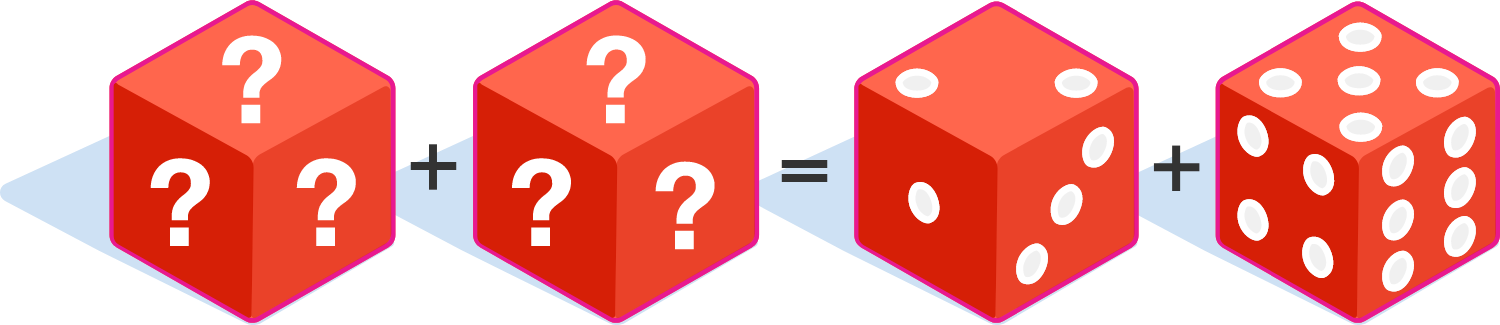# The Diciest Challenge

Probability Level 5We know that when rolling two standard 6-sided dice, each numbered 1 to 6, we can roll a sum of 2 with a chance of $\frac1{36}$, roll a sum of 3 with a chance of $\frac2{36}$, ... , and roll a sum of 12 with a chance of $\frac1{36}$.

Now you are given two blank 6-sided fair dices; prove that there is one other way to label the two dice with not necessarily distinct positive integers of your choice in such a way that we get the exact same probability for each sum as when rolling the two standard 6-sided dice shown above.

Let the numbers on the faces of the first dice be denoted as $a_1, a_2, a_3, a_4, a_5, a_6$, where $a_1 \leq a_2 \leq a_3 \leq a_4 \leq a_5 \leq a_6$.

Similarly, let the numbers on the faces of the second dice be denoted as $b_1, b_2, b_3, b_4, b_5, b_6$, where $b_1 \leq b_2 \leq b_3 \leq b_4 \leq b_5 \leq b_6$ and $a_6 < b_6$.

Submit your answer the concatenation of these 12 digits, $\overline{a_1 a_2 a_3 a_4 a_5 a_6 b_1 b_2 b_3 b_4 b_5 b_6 }$.

For example, if the respective faces of the two dices that you've found are $(2,3,3,6,6,7)$ and $(1,4,5,6,6,9)$, submit your answer as 233667145669.

×